# SSAT Upper Level Math : SSAT Upper Level Quantitative (Math)

## Example Questions

### Example Question #4 : How To Find The Properties Of An Exponent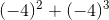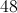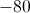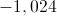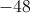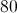Explanation: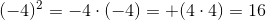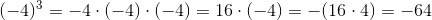Therefore,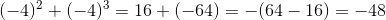### Example Question #1 : How To Find The Properties Of An Exponent

Express 0.00000000000097 in scientific notation.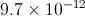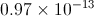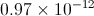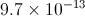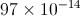Explanation:

To rewrite a very small number in scientific notation:

Write the number.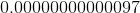Move the decimal point right as many places as needed until it follows the first nonzero digit, which here is the nine. Count the number of places it is moved - here it will be thirteen places.

The number formed is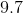, which will be placed in front;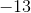, the negative of the number of places counted, will be the exponent. The number, in scientific notation, will be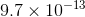.

### Example Question #1 : How To Find The Properties Of An Exponent

Evaluate: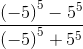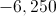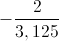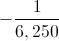The quantity is undefined.

The quantity is undefined.

Explanation: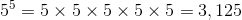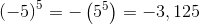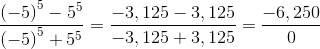This is an undefined quantity.

### Example Question #11 : Generate Equivalent Numerical Expressions: Ccss.Math.Content.8.Ee.A.1

Evaluate: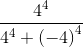The quantity is undefined.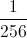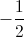Explanation: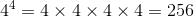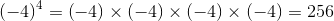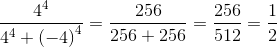### Example Question #2 : How To Find The Properties Of An Exponent

Evaluate: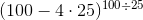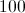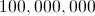The expression is undefined.Explanation: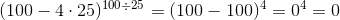as 0 taken to any positive power is equal to 0.

### Example Question #11 : Properties Of Exponents

Evaluate: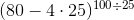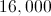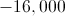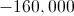The expression is undefined.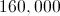Explanation: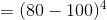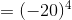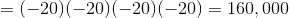### Example Question #11 : How To Find The Properties Of An Exponent

If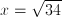then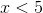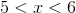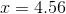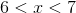Explanation: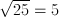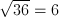34 is between 25 and 36, thus its square root must be between the square roots of 25 and 36.  x must be a number between 5 and 6.

### Example Question #12 : How To Find The Properties Of An Exponent

Express the result in scientific notation: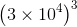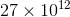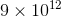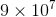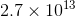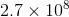Explanation:

Applying the power of a product property of exponents, then the power of a power property: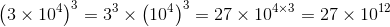This is not in scientific notation, so we adjust it as follows, applying the product of powers property: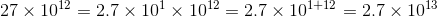### Example Question #13 : How To Find The Properties Of An Exponent

Assume all variables to be nonzero.

Simplify: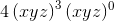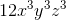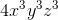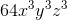Explanation:

Since any nonzero expression taken to the power of 0 is equal to 1,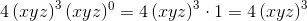Using the power of a product property of exponents, this becomes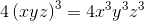### Example Question #14 : How To Find The Properties Of An Exponent

Express the result in scientific notation: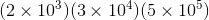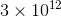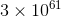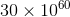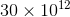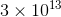Explanation:

Apply the product of powers property: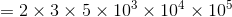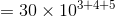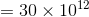Since this is not in scientific notation, adjust as follows: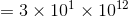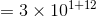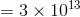### All SSAT Upper Level Math Resources